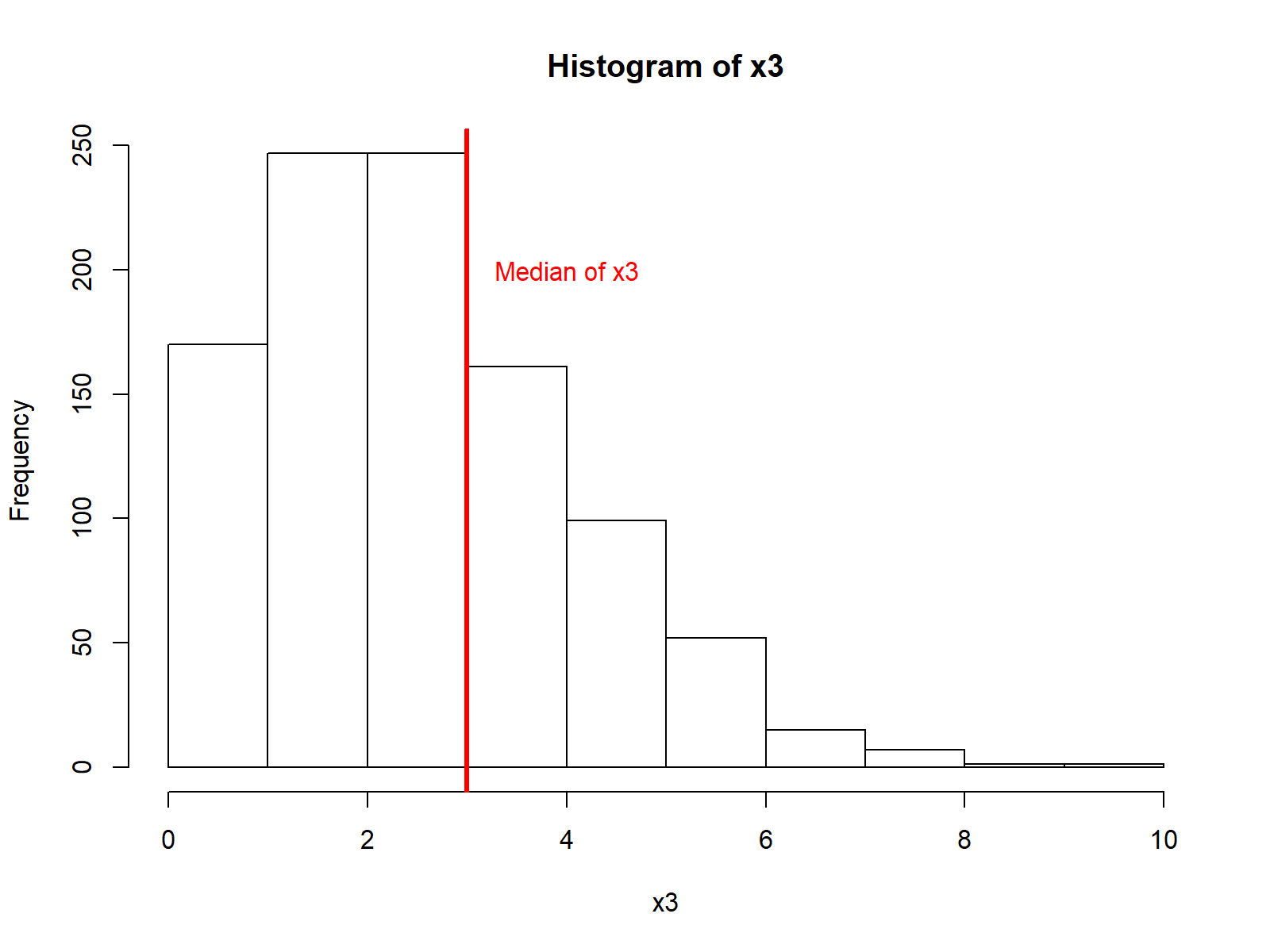# Median in R (5 Examples)

This tutorial shows how to compute the median in R.

The article is mainly based on the median() function. So let’s have a look at the basic R syntax and the definition of the median function first:

Basic R Syntax of median():

`median(x)`

Definition of median():

The median R function computes the sample median of a numeric input vector.

The R tutorial contains five examples for the application of the median function in R.

Let’s dive in!

## Example 1: Basic Application of median() in R

Before we can apply the median function, we need to create some example data. Consider the following numeric vector:

`x1 <- c(8, 5, 3, 7, 8, 1, 6, 5)      # Create example vector`

We can now use the median R function to compute the median of our example vector:

```median(x1)                           # Apply median function
# 5.5```

As you can see based on the RStudio console output, the median of our example vector is 5.5.

Note: Our example vector has an even length, resulting in a median value that does not exist in our example vector. The value 5.5 is the mean of the two middle values (i.e. 5 and 6). Learn more about the concept of even and uneven input vectors when calculating the median here.

## Example 2: Compute Median of Vector with NAs

A common issue in survey data is the occurrence of NAs (i.e. missing values). Let’s add such an NA value to our example vector:

`x2 <- c(8, 5, 3, 7, 8, 1, 6, 5, NA)  # Example vector with NA`

If we now apply the median R function to this vector, the RStudio console returns NA:

```median(x2)                           # Apply median function
# NA```

Fortunately, the median function provides the option na.rm, which enables the user to exclude all NA values before the computation of the median:

```median(x2, na.rm = TRUE)             # median function with na.rm
# 5.5```

## Example 3: Median of Column in Real Data Set

We can also compute the median of a column of a data matrix. For this example, I’m going to use the Iris Flower data. Let’s load and inspect the data structure:

```data(iris)                           # Load iris dataTable 1: First 6 Rows of Iris Data Matrix.

We can apply the median function to the first column of this data frame as follows:

```median(iris\$Sepal.Length)            # Median of first column
# 5.8```

The median of the column Sepal.Length is 5.8.

Note: We can also calculate the median across all columns and rows of our data with the colMedians and colRows functions. You can learn more about the function in this R programming tutorial.

## Example 4: Median by Group

The Iris Flower data set also contains a group indicator (i.e. the column Species). We can combine the aggregate function with the median function to get the median by group:

```aggregate(iris\$Sepal.Length,         # Median by group
list(iris\$Species),
median)
#    Group.1   x
#     setosa 5.0
# versicolor 5.9
#  virginica 6.5```

The group setosa has a median of 5.0; the group versicolor has a median of 5.9; and the group virginica has a median of 6.5.

## Example 5: Visualize Median in Boxplot & Histogram

In statistical research, the median is often used in figures and graphics. Let’s create another example vector with larger sample size to get some nicer looking graphs:

```set.seed(1717)                       # Set seed
x3 <- rpois(1000, 3)                 # Create larger example vector```

Medians are typically visualized in boxplots, as you can see based on the following R syntax and Figure 1:

```boxplot(x3)                          # Boxplot with median
text(x = 1, y = 3.25,                # Add text to boxplot
"Median of x3",
col = "red")```Figure 1: Boxplot with Median in R.

However, we could also draw a median line to other types of plots such as barcharts, scatterplots, histograms and so on. The following R code and Figure 2 show a median line drawn to a histogram:

```hist(x3)                             # Histogram in R
abline(v = median(x3),               # Add median to histogram
col = "red",
lwd = 3)```

text(x = 5, y = 200, # Add text to histogram
“Median of x3”,
col = “red”)Figure 2: Histogram with Vertical Median Line in R.

## Video, Further Resources & Summary

This tutorial illustrated how to use the median in different data scenarios in the R programming language.

In case you need further explanations on the code of this page, you may check out the following YouTube video on the Statistics Globe YouTube channel. It explains the syntax of this article in some more detail:

Please accept YouTube cookies to play this video. By accepting you will be accessing content from YouTube, a service provided by an external third party.If you accept this notice, your choice will be saved and the page will refresh.

However, in case you want to know other stuff about the theoretical research concept of the median, you could have a look at the following YouTube video of the mathantics channel.

The speaker of the video does not only explain the median, but also of the related concepts of the mode and mean. If you are not sure which metric to use for the calculation of an average, this video may be a good help.

Please accept YouTube cookies to play this video. By accepting you will be accessing content from YouTube, a service provided by an external third party.If you accept this notice, your choice will be saved and the page will refresh.

Furthermore, you might want to have a look at some of the other R tutorials of this website:

I hope that you at this point know how to use the median command in R. However, if you have any additional questions or comments, don’t hesitate let me know in the comments section below. In addition, you might want to subscribe to my email newsletter for more R tutorials.

Subscribe to the Statistics Globe Newsletter